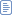#2006年11月2日

匹配中文字符的正则表达式： [\u4e00-\u9fa5]

String.prototype.len=function(){return this.replace([^\x00-\xff]/g,"aa").length;}

String.prototype.trim = function()
{
return this.replace(/(^\s*)|(\s*\$)/g, "");
}

function IP2V(ip)
{
re=/(\d+)\.(\d+)\.(\d+)\.(\d+)/g //匹配IP地址的正则表达式
if(re.test(ip))
{
return RegExp.\$1*Math.pow(255,3))+RegExp.\$2*Math.pow(255,2))+RegExp.\$3*255+RegExp.\$4*1
}
else
{
throw new Error("Not a valid IP address!")
}
}

var ip="10.100.20.168"
ip=ip.split(".")

var s="abacabefgeeii"
var s1=s.replace(/(.).*\1/g,"\$1")
var re=new RegExp("["+s1+"]","g")
var s2=s.replace(re,"")

s="http://www.9499.net/page1.htm"
s=s.replace(/(.*\/){0,}([^\.]+).*/ig,"\$2")

MTracer 下载
posted @ 2006-11-16 15:46 展昭 阅读(755) | 评论 (1)编辑 收藏

public int compareTo(Object o)

public int compareTo(Object o) {
DbPoJo po = null;
if(o instanceof DbPoJo)
po = (DbPoJo)o;
return po.getId().compareTo(this.getId());
}

Collections.sort(returnList);

posted @ 2006-11-02 12:16 展昭 阅读(2000) | 评论 (3)编辑 收藏